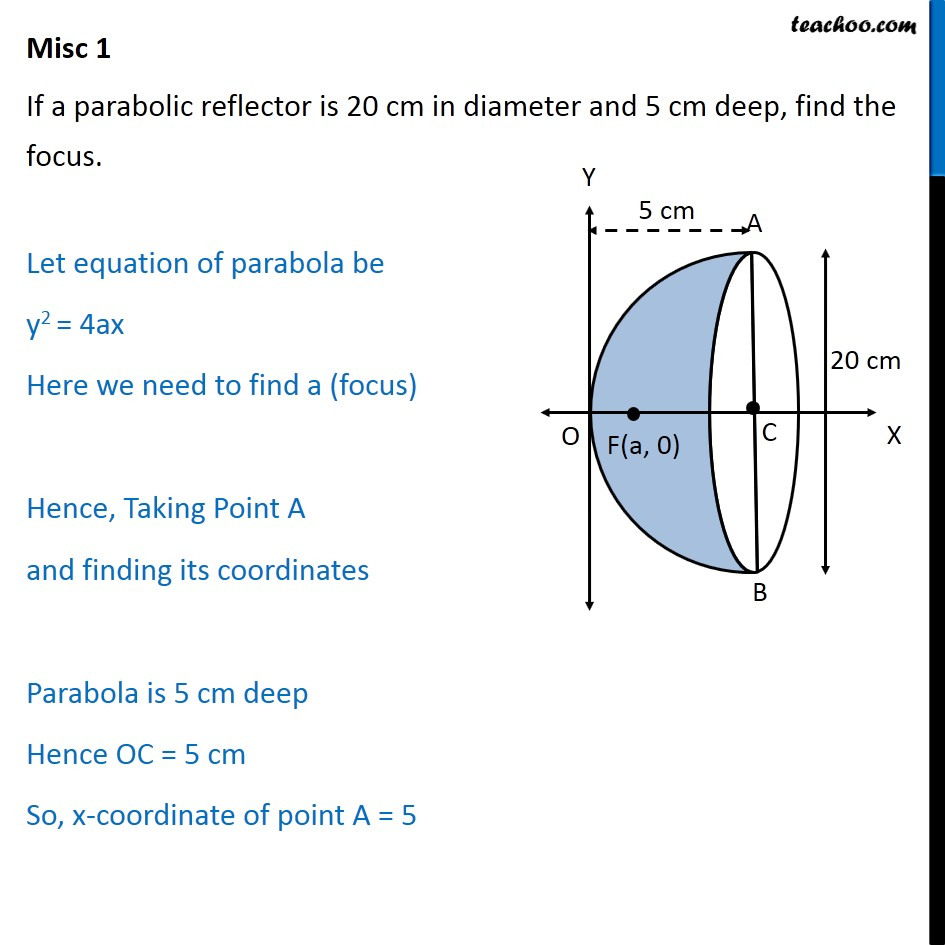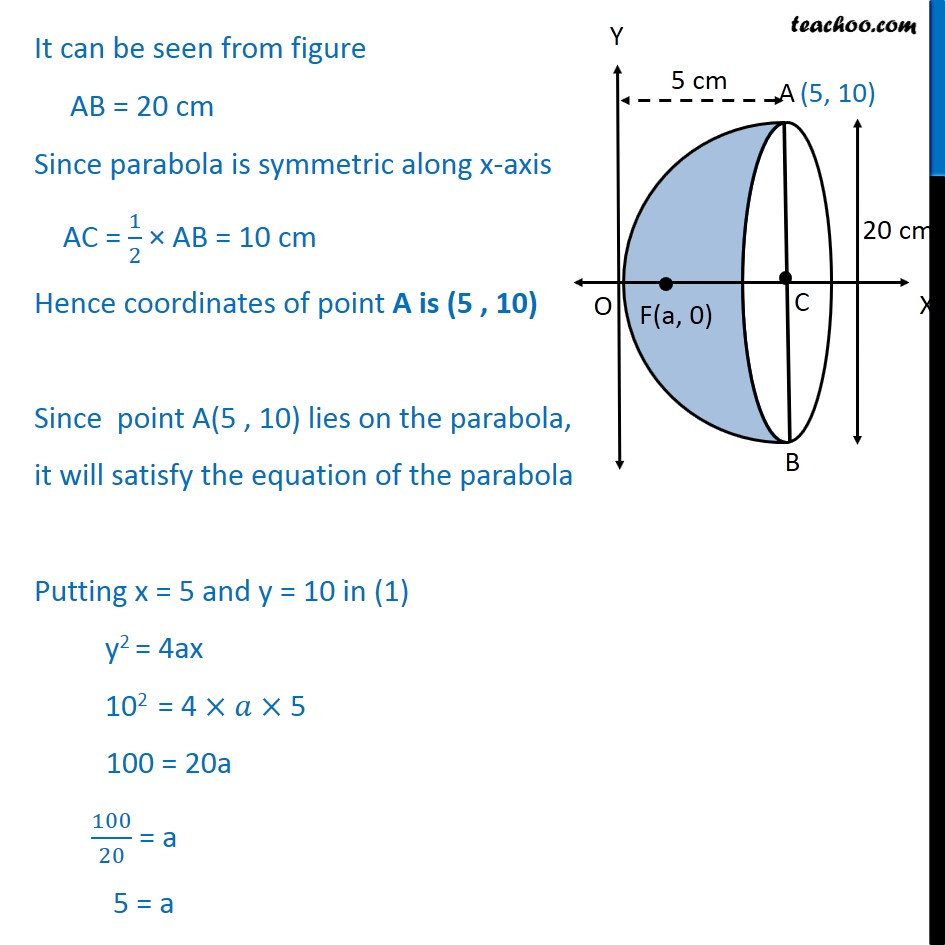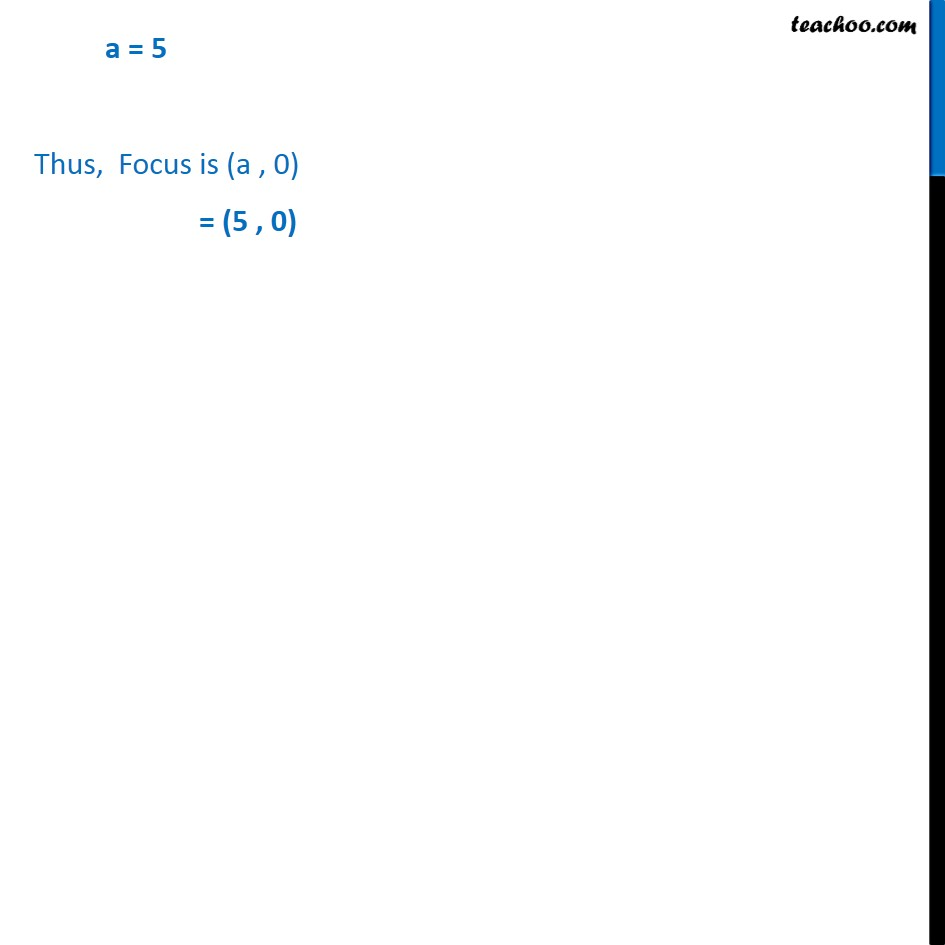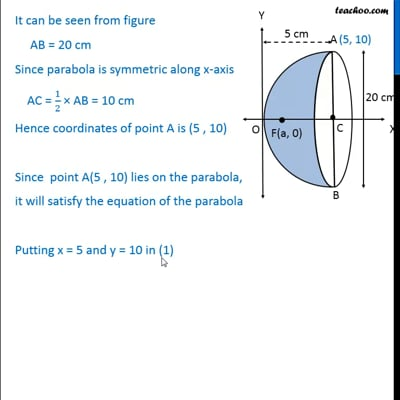Miscellaneous

Chapter 11 Class 11 Conic Sections
Serial order wiseThis video is only available for Teachoo black users

Solve all your doubts with Teachoo Black (new monthly pack available now!)

### Transcript

Misc 1 If a parabolic reflector is 20 cm in diameter and 5 cm deep, find the focus. Let equation of parabola be y2 = 4ax Here we need to find a (focus) Hence, Taking Point A and finding its coordinates Parabola is 5 cm deep Hence OC = 5 cm So, x-coordinate of point A = 5 It can be seen from figure AB = 20 cm Since parabola is symmetric along x-axis AC = 1 2 AB = 10 cm Hence coordinates of point A is (5 , 10) Since point A(5 , 10) lies on the parabola, it will satisfy the equation of the parabola Putting x = 5 and y = 10 in (1) y2 = 4ax 102 = 4 5 100 = 20a 100 20 = a 5 = a a = 5 Thus, Focus is (a , 0) = (5 , 0)